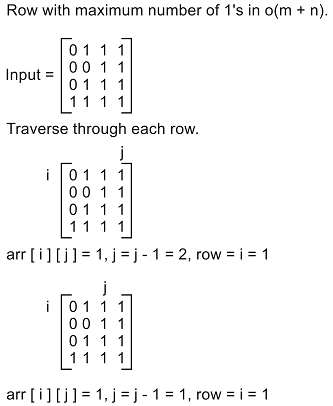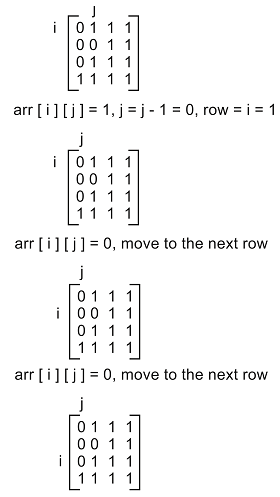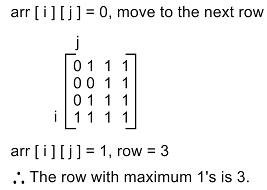# Find the row with maximum number of 1's

## Given a matrix(2D array) containing binary digits with each row sorted, this function will find the row which as maximum number of 1's .

### Example

INPUT:
0 1 1 1
0 0 1 1
0 1 1 1
1 1 1 1

OUTPUT:
3

In the above example, third row contains maximum number of 1's ie, four 1's

Time Complexity: O(mlogn),

where m is the number of rows and n is the number of columns

In this method, the main idea is to do binary search in each row and find the index where 1 has occured for the first time. The output is the row which has the least index value.

## Algorithm

1. Traverse through all the rows in the matrix

2. For each row, do binary search and find the index of the first occurrence of the 1 in that row
Binary search
a. If the mid element is 1 and mid-1 element is 0 then return mid index
b. If the mid element is 0, then do binary search on the right half ie, from mid+1 to n
c. else, do binary search on left half ie, from 0 to mid-1.

3. Return the row which has the least index.

## C++ Program

``````#include <bits/stdc++.h>
#include <climits>
#define m 4
#define n 4
using namespace std;

//This function will return the index of the first one using binary search
int firstoneIndex(int arr[], int low, int high)
{
if (low <= high)
{
int mid = low + (high - low)/2;

if ((mid==0 || arr[mid -1]==0) && arr[mid]==1)
{
return mid;
}
else if(arr[mid]==0)
{
return firstoneIndex(arr, (mid+1), high);
}
else
{
return firstoneIndex(arr,low, (mid-1));
}
}
return -1;
}
//In this function we will compare all indexes of first one
//we will print the row which has the least index
int maxOnes(int arr[m][n])
{
int row = 0;
int index = INT_MAX;
for (int i = 0; i < m; ++i)
{
int first_index = firstoneIndex(arr[i],0,n-1);
if (first_index != -1 && first_index<index)
{
index = first_index;
row = i;
}
}
cout<<"The row having maximum ones is "<<row<<endl;

}
int main()
{
int arr[m][n] = {{0,1,1,1},{0,0,1,1},{1,1,1,1},{0,1,1,1}};
maxOnes(arr);
return 0;
}``````

Time Complexity: O(m+n)

## Algorithm

1. Traverse through all the rows in the matrix

2. Start from the right corner element in the first row, go left until you find a 0 and go to next row until you find a 1.

3. return the last row that you encounter.## C++ Program

``````#include <bits/stdc++.h>
#define m 4
#define n 4
using namespace std;
//In this function, we will go left until we find a 0 ie, j=j-1
//go to next row until we find a 1
int maxOnes(int arr[m][n])
{
int row = 0;
int index = INT_MAX;
int j = n -1 ;
for (int i = 0; i < m; ++i)
{
while(arr[i][j]==1 && j>=0)
{
j= j-1;
row = i;
}
}
cout<<"The row having maximum ones is "<<row<<endl;

}
int main()
{
int arr[m][n] = {{0,1,1,1},{0,0,1,1},{1,1,1,1},{0,1,1,1}};
maxOnes(arr);
return 0;
}``````

Scroll to Top